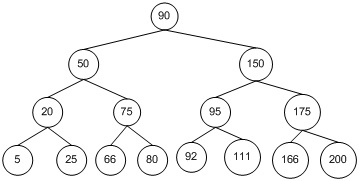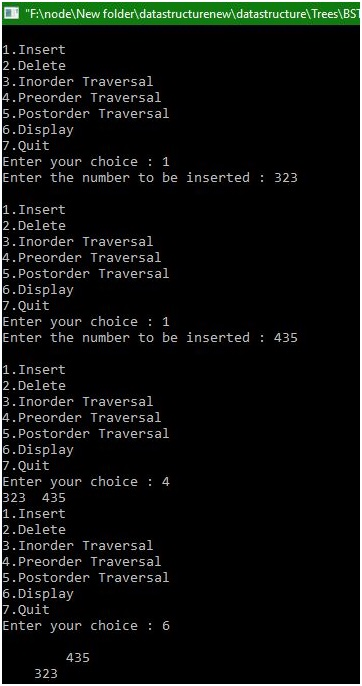# Insertion, Deletion and Traversal in Binary Search Tree

In this example, you will learn about what is Binary search tree (BST)? And C program for Insertion, Deletion, and Traversal in Binary Search Tree.
Submitted by Manu Jemini, on December 24, 2017

A Binary Search Tree (BST) is a widely used data structure. In that data structure, the nodes are in held in a tree-like structure. A Tree-like structure means a parent node is linked with its child nodes. In Binary Search tree a parent node can have only two child node. Nodes in a tree are linked together. The top node is called the root node or simply root.

The Value of parent node should be greater than the value of child node and smaller than equal to the value of right child node.

In the example below, a binary search tree has been implemented. The Tree facilitates you to insert new node and traverse it in various ways.Image source : https://i-msdn.sec.s-msft.com/dynimg/IC520.gif

In Pre-order the parent node visited first then the left child node and at last the right child node.

In In-order the left child node visited first then parent node and at last the right child node.

In Post-order the left child node visited first then the right child node and in last parent node.

## C program for Binary Search Tree (BST) implementation

```# include <stdio.h>
# include <malloc.h>

struct node
{
int info;
struct node *lchild;
struct node *rchild;
}*root;

void find(int item,struct node **par,struct node **loc)
{
struct node *ptr,*ptrsave;

if(root==NULL)  /*tree empty*/
{
*loc=NULL;
*par=NULL;
return;
}
if(item==root->info) /*item is at root*/
{
*loc=root;
*par=NULL;
return;
}
/*Initialize ptr and ptrsave*/
if(item<root->info)
ptr=root->lchild;
else
ptr=root->rchild;
ptrsave=root;

while(ptr!=NULL)
{
if(item==ptr->info)
{       *loc=ptr;
*par=ptrsave;
return;
}
ptrsave=ptr;
if(item<ptr->info)
ptr=ptr->lchild;
else
ptr=ptr->rchild;
}/*End of while */
*par=ptrsave;
}/*End of find()*/

void insert(int item)
{       struct node *tmp,*parent,*location;
find(item,&parent,&location);
if(location!=NULL)
{
return;
}

tmp=(struct node *)malloc(sizeof(struct node));
tmp->info=item;
tmp->lchild=NULL;
tmp->rchild=NULL;

if(parent==NULL)
root=tmp;
else
if(item<parent->info)
parent->lchild=tmp;
else
parent->rchild=tmp;
}/*End of insert()*/

void case_a(struct node *par,struct node *loc )
{
if(par==NULL) /*item to be deleted is root node*/
root=NULL;
else
if(loc==par->lchild)
par->lchild=NULL;
else
par->rchild=NULL;
}/*End of case_a()*/

void case_b(struct node *par,struct node *loc)
{
struct node *child;

/*Initialize child*/
if(loc->lchild!=NULL) /*item to be deleted has lchild */
child=loc->lchild;
else                /*item to be deleted has rchild */
child=loc->rchild;

if(par==NULL )   /*Item to be deleted is root node*/
root=child;
else
if( loc==par->lchild)   /*item is lchild of its parent*/
par->lchild=child;
else                  /*item is rchild of its parent*/
par->rchild=child;
}/*End of case_b()*/

void case_c(struct node *par,struct node *loc)
{
struct node *ptr,*ptrsave,*suc,*parsuc;

/*Find inorder successor and its parent*/
ptrsave=loc;
ptr=loc->rchild;
while(ptr->lchild!=NULL)
{
ptrsave=ptr;
ptr=ptr->lchild;
}
suc=ptr;
parsuc=ptrsave;

if(suc->lchild==NULL && suc->rchild==NULL)
case_a(parsuc,suc);
else
case_b(parsuc,suc);

if(par==NULL) /*if item to be deleted is root node */
root=suc;
else
if(loc==par->lchild)
par->lchild=suc;
else
par->rchild=suc;

suc->lchild=loc->lchild;
suc->rchild=loc->rchild;
}/*End of case_c()*/
int del(int item)
{
struct node *parent,*location;
if(root==NULL)
{
printf("Tree empty");
return 0;
}

find(item,&parent,&location);
if(location==NULL)
{
printf("Item not present in tree");
return 0;
}

if(location->lchild==NULL && location->rchild==NULL)
case_a(parent,location);
if(location->lchild!=NULL && location->rchild==NULL)
case_b(parent,location);
if(location->lchild==NULL && location->rchild!=NULL)
case_b(parent,location);
if(location->lchild!=NULL && location->rchild!=NULL)
case_c(parent,location);
free(location);
}/*End of del()*/

int preorder(struct node *ptr)
{
if(root==NULL)
{
printf("Tree is empty");
return 0;
}
if(ptr!=NULL)
{
printf("%d  ",ptr->info);
preorder(ptr->lchild);
preorder(ptr->rchild);
}
}/*End of preorder()*/

void inorder(struct node *ptr)
{
if(root==NULL)
{
printf("Tree is empty");
return;
}
if(ptr!=NULL)
{
inorder(ptr->lchild);
printf("%d  ",ptr->info);
inorder(ptr->rchild);
}
}/*End of inorder()*/

void postorder(struct node *ptr)
{
if(root==NULL)
{
printf("Tree is empty");
return;
}
if(ptr!=NULL)
{
postorder(ptr->lchild);
postorder(ptr->rchild);
printf("%d  ",ptr->info);
}
}/*End of postorder()*/

void display(struct node *ptr,int level)
{
int i;
if ( ptr!=NULL )
{
display(ptr->rchild, level+1);
printf("\n");
for (i = 0; i < level; i++)
printf("    ");
printf("%d", ptr->info);
display(ptr->lchild, level+1);
}/*End of if*/
}/*End of display()*/
main()
{
int choice,num;
root=NULL;
while(1)
{
printf("\n");
printf("1.Insert\n");
printf("2.Delete\n");
printf("3.Inorder Traversal\n");
printf("4.Preorder Traversal\n");
printf("5.Postorder Traversal\n");
printf("6.Display\n");
printf("7.Quit\n");
scanf("%d",&choice);

switch(choice)
{
case 1:
printf("Enter the number to be inserted : ");
scanf("%d",&num);
insert(num);
break;
case 2:
printf("Enter the number to be deleted : ");
scanf("%d",&num);
del(num);
break;
case 3:
inorder(root);
break;
case 4:
preorder(root);
break;
case 5:
postorder(root);
break;
case 6:
display(root,1);
break;
case 7:
break;
default:
printf("Wrong choice\n");
}/*End of switch */
}/*End of while */
}/*End of main()*/
```

Output